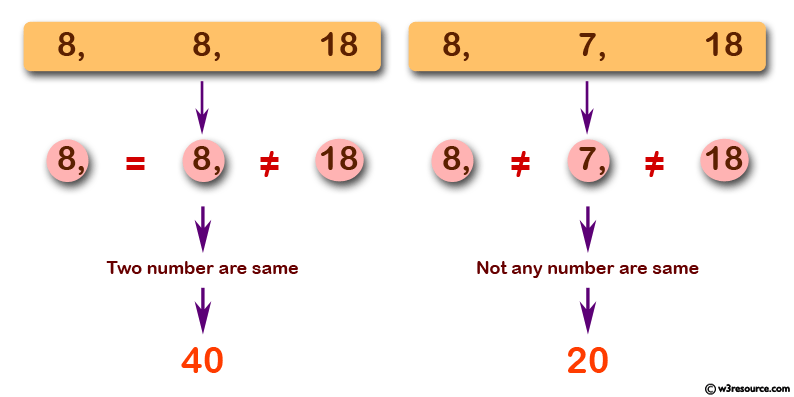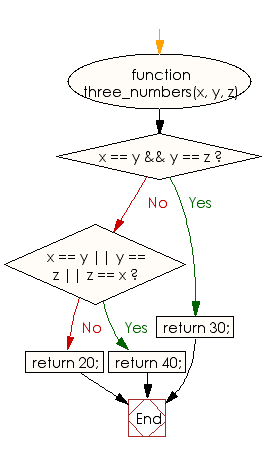# JavaScript: Check three given numbers, if the three numbers are same return 30 otherwise return 20 and if two numbers are same return 40

## JavaScript Basic: Exercise-41 with Solution

Write a JavaScript program to check three given numbers, if the three numbers are same return 30 otherwise return 20 and if two numbers are same return 40.

Pictorial Presentation:Sample Solution:

HTML Code:

``````<!DOCTYPE html>
<html>
<meta charset="utf-8">
<meta name="viewport" content="width=device-width">
<title>JavaScript program to check three given numbers, if the three numbers are same return 30 otherwise return 20 and if two numbers are same return 40.</title>
<body>

</body>
</html>
```
```

JavaScript Code:

``````function three_numbers(x, y, z) {
if (x == y && y == z) {
return 30;
}

if (x == y || y == z || z == x) {
return 40;
}

return 20;
}
console.log(three_numbers(8, 8, 8));
console.log(three_numbers(8, 8, 18));
console.log(three_numbers(8, 7, 18));
```
```

Sample Output:

```30
40
20
```

Flowchart:ES6 Version:

``````function three_numbers(x, y, z) {
if (x == y && y == z) {
return 30;
}

if (x == y || y == z || z == x) {
return 40;
}

return 20;
}
console.log(three_numbers(8, 8, 8));
console.log(three_numbers(8, 8, 18));
console.log(three_numbers(8, 7, 18));
``````

Live Demo:

See the Pen JavaScript: Check three given numbers - basic-ex-41 by w3resource (@w3resource) on CodePen.

Improve this sample solution and post your code through Disqus

What is the difficulty level of this exercise?

Test your Programming skills with w3resource's quiz.

﻿

## JavaScript: Tips of the Day

Checks if a string is an anagram of another string (case-insensitive, ignores spaces, punctuation and special characters)

Example:

```const isAnagram = (str1, str2) => {
const normalize = str =>
str
.toLowerCase()
.replace(/[^a-z0-9]/gi, '')
.split('')
.sort()
.join('');
return normalize(str1) === normalize(str2);
};
console.log(isAnagram('iceman', 'cinema')); // true
```

Output:

```true
```